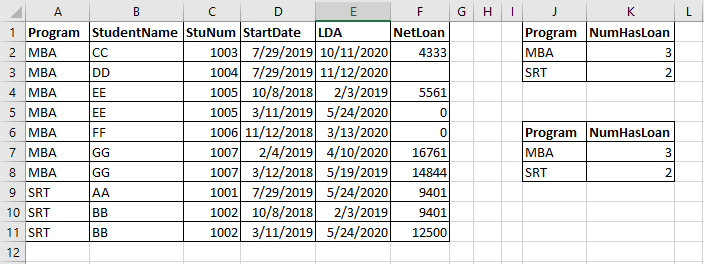Excel: How to Unduplicated Count Number of Students in the Program

For example, an Excel Worksheet below, count unduplicated number of students have loans in the program. Note: NetLoan Column may have blank or 0 amount.If we named Column A as Program, Column B as StudentName, and Column F as NetLoan already to simplify formula later, then the formula in K2 is below:

= IFERROR(ROWS(UNIQUE(FILTER(StuNum,(TRIM(Program)=TRIM(J2)) *(NetLoan>0)))),0)

If we did not name the Columns, the formula in K7 is below:

= IFERROR(ROWS(UNIQUE(FILTER(\$C:\$C,(TRIM(\$A:\$A)=TRIM(J7)) *(\$F:\$F>0)))),0)